# Worksheet on Linear Inequations | Solving Linear Inequalities Worksheet with Answers

Get Worksheet on Linear Inequations from this page. Free Linear Inequalities Worksheet has different questions and solutions along with the detailed explanation. Refer Linear Inequations and Linear Inequalities Worksheet to get solutions for all inequation problems. We have given problems on each and every concept individually.

Students who are seriously concentrating on exams can go through the Linear Inequations Worksheet and practice questions. Solve all the questions mentioned below to gain knowledge and confidence to attend the examination. This page also helps you to score maximum marks in the exam.

1. Write the word statement for each of the following inequations:

(a) x ≥ 8

(b) x < -2

(c) x > 6

(d) x ≤ 5

Solution:

(a) x ≥ 8

The variable x is greater than equal to 8. The possible values of x are 8 and more than 8.

(b) x < -2

The variable x is less than -2. The possible values of x are -1 and less than it.

(c) x > 6

The variable x is greater than 6. The possible values of x are 7, 8, and so on.

(d) x ≤ 5

The variable x is less than and equal to 5. The possible values of x are 5, 4, 3, 2, 1 and so on.

2. Write the resulting equation in each of the following cases when each side of the equation:

(a) x < 2 increased by 2.

(b) x > 4 decreased by 7.

(c) x ≤ 6 multiplied by 4.

(d) x ≥ 25 divided by 5.

Solution:

(a) x < 2 increased by 2.

Add 2 to both sides of inequation.

x + 2 < 2 + 2

x + 2 < 4.

(b) x > 4 decreased by 7.

Subtract 7 from both sides of the inequation

x – 7 > 4 – 7

x – 7 > -3

(c) x ≤ 6 multiplied by 4.

x x 4 ≤ 6 x 4

4x ≤ 24

(d) x ≥ 25 divided by 5.

x/5 ≥ 25/5

x/5 ≥ 5

3. Write the word statement for the following linear inequations:

(a) x ≤ -4

(b) x > 9

(c) x < -1

Solution:

(a) x ≤ -4

The variable x is less than and equal to -4. The possible values of x are -4, -5, -6, and so on.

(b) x > 9

The variable x is greater than 9. The possible values of x are 10, 11, 12, and so on.

(c) x < -1

The variable x is less than -1. The possible values of x are -2, -3, -4, -5, -6, and so on.

4. Write the resulting inequation for each question:

(a) x < 5 multiplied by 2.

(b) x > 6 divided by 3.

(c) x ≥ 10 increased by 5.

Solution:

(a) x < 5 multiplied by 2.

Multiply 2 by both sides.

x x 2 < 5 x 2

2x < 10.

(b) x > 6 divided by 3.

Divide 3 by each side

x/3 > 6/3

x/3 > 2.

(c) x ≥ 10 increased by 5.

x + 5 ≥ 10 + 5

x + 5 ≥ 15.

5. Draw the separate number line for the ‘following inequations’.

(a) x < -9, x ∈ I

(b) x ≥ 7, x ∈ N

(c) x > 8, x ∈ W

Solution:

(a) x < -9, x ∈ I

Replacement set = {. . ., -4, -3, -2, -1, 0, 1, 2, 3, 4, 5, . . .}

Solution set for given inequation S = {. . . -12, -11, -10}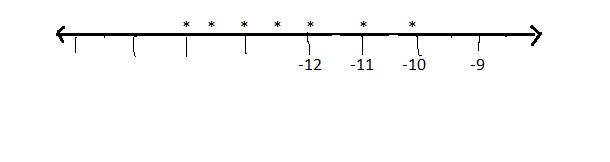(b) x ≥ 7, x ∈ N

Replacement set = {1, 2, 3, 4, 5, . . .}

Solution set for given inequation S = {7, 8, 9, . . . }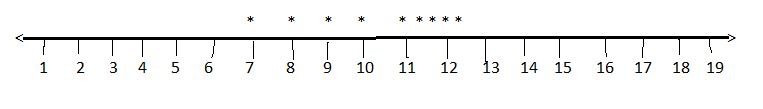(c) x > 8, x ∈ W

Replacement set = {0, 1, 2, 3, 4, 5, 6, . . .}

Solution set for given inequation S = {9, 10, 11, 12, 13, . . }6. Draw a separate number line for the inequation -5 < x ≤ 5  when (i) x ∈ I, (ii) x ∈ W, (iii) x ∈ N. Write the replacement set and solution set in each case.

Solution:

Given linear inequation is -5 < x ≤ 5

It has two cases.

Case I: -5 < x

Case II: x ≤ 5

(i) x ∈ I

Replacement set = {. . ., -4, -3, -2, -1, 0, 1, 2, 3, 4, 5, . . .}

Solution set for -5 < x is {-4, -3, -2, -1, 0, . . .} = P

Solution set for x ≤ 5 is {. . . 1, 2, 3, 4, 5} = Q

Therefore, required solution set S = P ∩ Q

= {-4, -3, -2, -1, 0, . . .} ∩ {. . . 1, 2, 3, 4, 5}

= {-4, -3, -2, -1, 0, 1, 2, 3, 4, 5}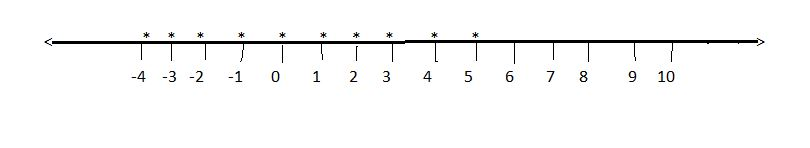(ii) x ∈ W

Replacement set = {0, 1, 2, 3, 4, 5, 6, . . .}

Solution set for -5 < x is {0, 1, 2, 3, 4, 5, 6, . . .} = P

Solution set for x ≤ 5 is {0, 1, 2, 3, 4, 5} = Q

Therefore, required solution set S = P ∩ Q

= {0, 1, 2, 3, 4, 5}(iii) x ∈ N

Replacement set = {1, 2, 3, 4, 5, . . .}

Solution set for -5 < x is {1, 2, 3, 4, 5, . . .} = P

Solution set for x ≤ 5 is {1, 2, 3, 4, 5} = Q

Therefore, required solution set S = P ∩ Q

= {1, 2, 3, 4, 5}7. Solve the following linear inequations.

(a) x – 6 < 4, x ∈ W

(b) 4x + 7 > 15, x ∈ N

(c) x/2 + 5 ≤ 6, x ∈ I

Solution:

(a) x – 6 < 4, x ∈ W

Add 6 to both sides of the linear inequation.

x – 6 + 6 < 4 + 6

x < 10

Replacement set = {0, 1, 2, 3, 4, 5, 6, . . .}

Solution set for x < 10 is {0, 1, 2, 3, 4, 5, 6, 7, 8, 9, 10}

(b) 4x + 7 > 15, x ∈ N

Subtract 7 from both sides of the inequation.

4x + 7 – 7 > 15 – 7

4x > 8

Divide each side of the inequation by 4.

4x/4 > 8/4

x > 2.

Replacement set = {1, 2, 3, 4, 5, . . .}

Solution set for x > 2 is {3, 4, 5, . . .}

(c) x/2 + 5 ≤ 6, x ∈ I

Subtract 5 from both sides of the inequation.

x/2 + 5 – 5 ≤ 6 – 5

x/2 ≤ 1

Multiply each side of the inequation by 2.

x/2 x 2 ≤ 1 x 2

x ≤ 2

Replacement set = {. . ., -4, -3, -2, -1, 0, 1, 2, 3, 4, 5, . . .}

Solution set for x ≤ 2 is {. . . -1, 0, 1, 2}

8. Solve the following inequations and represent them graphically.

(a) -x/3 > 5, x ∈ I

(b) 6x – 7 ≥ 17, x ∈ I

(c) -3x > 12, x ∈ I

Solution:

(a) -x/3 > 5

Multiply each side of the inequation by -3.

-x/3 x (-3) < 5 x (-3)

x < -15

Replacement set = {. . ., -4, -3, -2, -1, 0, 1, 2, 3, 4, 5, . . .}

Therefore, solution set for x > -15 is {. . . -17, -16}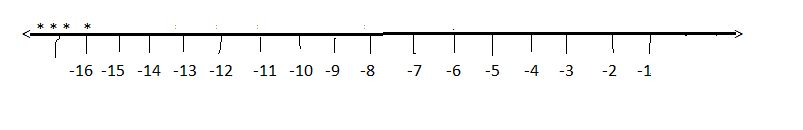(b) 6x – 7 ≥ 17

6x – 7 + 7 ≥ 17 + 7

6x ≥ 24

Divide each side by 6.

6x/6 ≥ 24/6

x ≥ 4

Replacement set = {. . ., -4, -3, -2, -1, 0, 1, 2, 3, 4, 5, . . .}

Therefore, solution set for x ≥ 4 is {4, 5, 6, 7, . . .}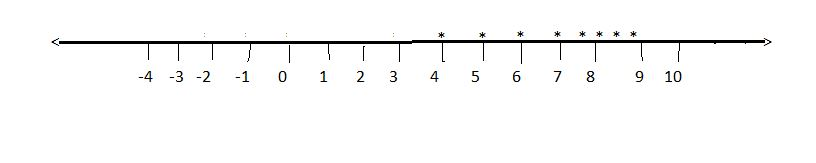(c) -3x > 12

Divide each side by -3.

-3x/(-3) > 12/(-3)

x < -4

Replacement set = {. . ., -4, -3, -2, -1, 0, 1, 2, 3, 4, 5, . . .}

Therefore, solution set for x < -4 is {. . . -8, -7, -6, -5}9. Compute the below mentioned inequations.

(a) x/2 > x/3 + 1, x ∈ W

(b) 3x + 5 < 4x – 6, x ∈ W

(c) x/6 – 1 ≥ 3, x ∈ W

(d) 8x – 9 > 9x + 10, x ∈ W

Solution:

(a) x/2 > x/3 + 1, x ∈ W

Move variable x to one side and constants to other side.

x/2 – x/3 > 1

L.C.M of 3, 2 is 6.

(3x – 2x) / 6 > 1

x/6 > 1

Divide each side by (6)

x/6 x (6) > 1 x (6)

x > 6

Replacement set = {0, 1, 2, 3, 4, 5, 6, . . .}

Therefore, solution set for x > 6 is {7, 8, 9, . .}

(b) 3x + 5 < 4x – 6, x ∈ W

Move variable x to one side and constants to other side.

5 – 6 < 4x – 3x

-1 < x

Replacement set = {0, 1, 2, 3, 4, 5, 6, . . .}

Therefore, solution set for -1 < x is {0, 1, 2, 3, 4, 5, 6, . . .}

(c) x/6 – 1 ≥ 3, x ∈ W

x/6 ≥ 3 + 1

x/6 ≥ 4

Multiply each side by 6.

x/6 x 6 ≥ 4 x 6

x ≥ 24

Replacement set = {0, 1, 2, 3, 4, 5, 6, . . .}

Therefore, solution set for x ≥ 24 is {24, 25, 26, . . .}

(d) 8x – 9 > 9x + 10, x ∈ W

Move variable x to one side and constants to other side.

-9 – 10 > 9x – 8x

-19 > x

Replacement set = {0, 1, 2, 3, 4, 5, 6, . . .}

Therefore, solution set for -19 > x is {0, 1, 2, 3, 4, 5, 6, . . .}

10. Represent the following linear inequations graphically.

(a) 7 – 3x < – 2, x ∈ N

(b) 8x – 5 ≥ 18, x ∈ N

(c) 5x/2 > 1, x ∈ N

Solution:

(a) 7 – 3x < – 2, x ∈ N

7 – 3x + 3x < -2 + 3x

7 < -2 + 3x

7 + 2 < -2 + 2 + 3x

9 < 3x

Divide each side by 3.

9/3 < 3x/3

3 < x

Replacement set = {1, 2, 3, 4, 5, . . .}

Therefore, solution set for 3 < x is {4, 5, 6, 7. . }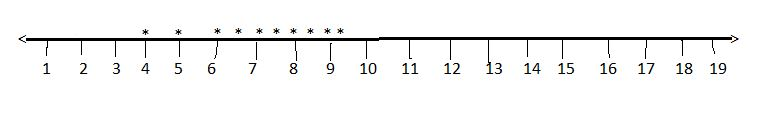(b) 8x – 5 ≥ 18, x ∈ N

8x – 5 + 5 ≥ 18 + 5

8x ≥ 23

Divide each side by 8.

8x/8 ≥ 23/8

x ≥ 2.87

Replacement set = {1, 2, 3, 4, 5, . . .}

Therefore, solution set for x ≥ 2.87 is {2.87, 3, 4, 5, . . }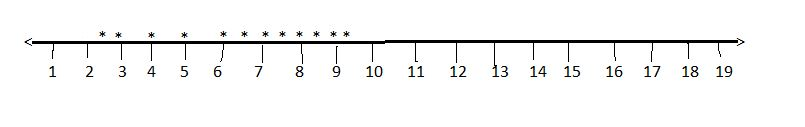(c) 5x/2 > 1, x ∈ N

Multiply each side by 2.

5x/2 x 2 > 1 x 2

5x > 2

Divide each side by 5.

5x/5 > 2/5

x > 0.4

Replacement set = {1, 2, 3, 4, 5, . . .}

Therefore, solution set for x > 0.4 is {1, 2, 3, 4, 5, . . .}